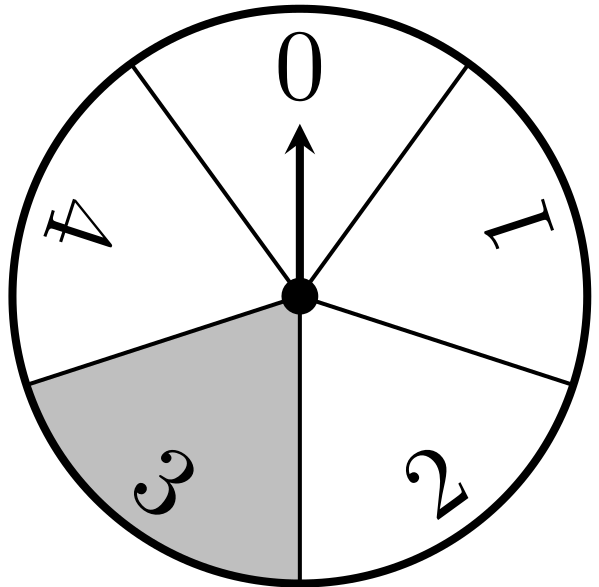시간 제한 메모리 제한 제출 정답 맞은 사람 정답 비율
1 초 512 MB 1 1 1 100.000%

## 문제

Katya has a younger brother Denis, who loves puzzles. It seemed to him that he had already solved all the puzzles in the world. But, one day, returning from school with Katya, he noticed a vending machine with a puzzle that he has never seen before. He asked Katya to play with this machine and win a new puzzle.Vending machine from the first example, before the start of the game.

The machine has a disk, divided into $n$ sectors, rotating arrow and a button. When the button is pressed, the arrow starts to spin, and the player receives this prize, corresponding to the sector on which it stops. After observing the machine, Katya noticed that it is quite primitive, and after each click of the button the arrow moves $k$ sectors clockwise. Also, Katya realized that the desired puzzle is at sector $x$, and it could be impossible to win. And then she asked you to help her.

Help Katya find out whether it is possible to win a puzzle, corresponding to sector $x$, if the arrow initially points to the sector $s$. And, if it is possible, calculate the minimal number of button clicks.

## 입력

A single line contains four integers $n$, $k$, $s$ and $x$ --- the number of sectors in the machine, number of sectors the arrow moves after one click, number of a sector the arrow points initially to, and the number of a sector corresponding to desired puzzle, respectively ($2 \leq n \leq 20\,000$; $1 \leq k < n$; $0 \leq s, x < n$; $x \neq s$).

## 출력

Output one integer, the minimal number of clicks you need to make to get the desired puzzle, or <<-1>>, if it is impossible.

## 서브태스크

번호 배점 제한
1 10

$k = 1$

2 15

$k = 2$

3 15

$s = 0$, $x = n-k$

4 30

It’s possible to get desired puzzle

5 30

No additional constraints.

## 예제 입력 1

5 2 0 3


## 예제 출력 1

4


## 예제 입력 2

6 3 1 3


## 예제 출력 2

-1


## 힌트

In the first example, the arrow will move through the sectors $0 \rightarrow 2 \rightarrow 4 \rightarrow 1 \rightarrow 3 \rightarrow \dots$. Thus, after $4$ button clicks you can get a puzzle from sector $3$. The figure in the statement shows the machine from the first example before the game starts.

In the second example, the arrow will move only between the two sectors: 1 and 4. So it's impossible to get puzzle from sector 3.

Notice, that samples do not satisfy the additional constraints of the first, second, third, and fourth subtasks. Your solution will run on tests of the first four subtasks even if it does not pass the sample tests.

## 채점 및 기타 정보

• 예제는 채점하지 않는다.